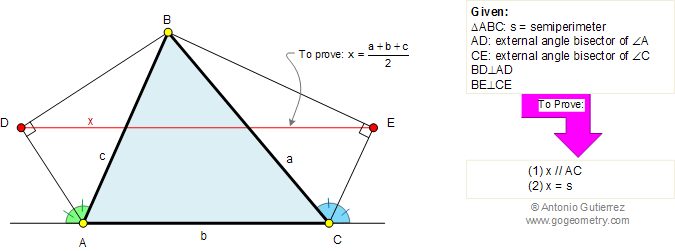Problem 384. Triangle, Angle bisector, Perpendicular, Parallel, Semiperimeter. Level: High School, SAT Prep, College geometry. The figure shows a triangle ABC with external angle bisectors AD and CE of angles A and C, respectively. BD is perpendicular to AD and BE is perpendicular to CE. Prove that (1) DE is parallel to AC, (2) DE is equal to the semiperimeter of triangle ABC.Problem 384 on Twitter: — José Luis da Vila (@jldavilaa01) August 10, 2017 Recent Additions